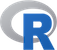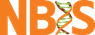@# Introduction

In this lab, we will go step-by-step through points that are necessary to create some nice-looking plots.

# Generating data

First, we will produce some random data that we will later plot. Make a data frame with

• 20 random coordinates (x,y): y coming from N(0,1) – normal distribution with mean=0 and sd=1
• radius r for each data point, r coming from N(0,1).
• The x coord takes random values from 1 to 25 and
• Each point (row of the data frame) has a name ind1 … ind25,

First, look at the defaults:

• plot the data in the simplest possible way.Click to see how
```
#20 random datapoints
x <- sample(c(1:25), size=20, replace=T)
y <- rnorm(n=20, mean=0, sd=1) # sample from normal
r <- rnorm(n=20, mean=0, sd=1) # radius from normal
names <- paste("ind", 1:20, sep="") # assign some names
data <- data.frame(cbind(X=x,Y=y, R=r), row.names=names)
plot(data[,1:2], cex=data\$R)
```

# Generating plot step-by-step

As you see, the points are displayed in a simple way, axes are set automatically, the radius is not reflected on the plot in any way (3rd dimension).

• build the plot from scratch, begin by displaying no points.Click to see how
```
plot(data[,1:2], type='n')

```

• we still got a box around the plot and axes, we do not want these either, remove these elements from the previous plot.Click to see how
```
plot(data[,1:2], type='n',xaxt='n', yaxt='n',
xlab="", ylab="", frame.plot=F)
```

• create X and Y axis so that they cover the whole range of x and y. Make axis text slightly smaller, 70% of the default. For the Y axis, set 10 equidistant tickmarks and set labels to their values rounded to two decimals. Turn the labels, so that they are parallel to the OX axis.Click to see how
```
#Create X axis
coords.x <- seq(min(data\$X),max(data\$X), by=1)
axis(side=1, # 1-bottom, 2-left, 3-top, 4-right
at=coords.x, # coordinates for tickmarks
cex.axis=.7 # make labels smaller
)
#Create Y axis
#we want 10 tickmarks along the data range
coords.y <- seq(min(data\$Y), max(data\$Y), length.out=10)
#and our labels will be the rounded values of y
labels.y <- round(coords.y, digits=2)
axis(side=2,
at=coords.y,
labels=labels.y, # we want specific labels
las=2 # turn the text so it is parallel to OX
)
```

• plot auxiliary lines (a grid) so that it is easier to read the plot. There should be a grey dashed line from each tickmark on both axes.Click to see how
```
abline(v=coords.x, col="darkgrey", lty=3)
abline(h=coords.y, col="darkgrey", lty=3)
#you could also use grid()
```

• define a new mycol function that takes a color name and a transparency value as two arguments and returns the corresponding rgb color value. OPTIONAL – if it seems to difficult, look up the answer.Click to see how
```
#Function for adding transparency to a given color.
mycol <- function(colname="olivedrab", transparency=.5) {
#convert color name to its RGB value and add the desired
#transparency
color <- c(as.vector(col2rgb(colname))/255, transparency)
# and make a new color from the above
color <- rgb(color, color, color, color)
return(color)
}
```

• plot datapoints so that their size is proportional to e^r (e to the power of r , e is the base of the natural logarithm and e=2.71…) where \$r\$ is the radius, points at even X should be round and blue and points at odd X square and grey.Click to see how
```
points(data[data\$X%%2 == 0,], pch=19,
cex=exp(r), col=mycol("slateblue", .5))
points(data[data\$X%%2 != 0,], pch=15,
cex=exp(r), col=mycol("grey", .5))
```

• plot centers of the points as a cross: grey for blue/even points and red for grey/odd points.Click to see how
```
points(data[data\$X%%2 == 0,], pch=3, cex=1, col="darkgrey")
points(data[data\$X%%2 != 0,], pch=3, cex=1, col="red")
```

• add grey text ‘Center’ at the center of the plot.Click to see how
```
center.x <- mean(range(data[,1]))
center.y <- mean(range(data[,2]))
text(x=center.x, y=center.y, "Center", col="lightgrey")
```

• add title ‘Odds and Ends’ and text ‘X’ and ‘Y’ on the margins of the appropriate axes.Click to see how
```
title("Odds and Ends")
mtext("Y", side=2, line=3, cex.lab=1,las=2, col="blue")
mtext("X", side=1, line=3, cex.lab=1,las=1, col="blue")
```

• add a legend for ‘odd’ and ‘even’ points. Place it in the top-right corner.Click to see how
```
legend('topright',
legend=c("odd", "even"),
col=c(mycol("slateblue", .5), mycol("grey", .5)),
pch=c(19,15),
cex=1,
pt.cex=1.2,
title="Legend",
bty='n'
)
```

# Visualizing baby growth data on a WHO centile grid

A female child was measured at the following dates:

• ‘30-09-2015’, ‘12-10-2015’, ‘19-10-2015’, ‘26-10-2015’, ‘07-11-2015’, ‘16-11-2015’, ‘30-11-2015’, ‘11-01-2016’, ‘08-02-2016’, ‘14-03-2016’, ‘05-04-2016’, ‘14-04-2016’, ‘31-05-2016’, ‘14-07-2016’,

• the measured weights in grams were: 3300, 3540, 3895, 4070, 4230, 4385, 4855, 5865, not taken, 6736, 7065, 7080, 7530, 7640 and
• the measured lengths: 43, no measurement taken, 53, 54, 55, 56, 58, 62.5, 65, 67, 67.5, 67.5, 70.5, 71.5.
• The headcircumference for the same datapoints was (in cm): 34, 35.5, 36.1, 36.8, 36.8, 37.3, 38, 40.2, 41.4, 42.1, not taken, 43, 44, 45.

Your task is to plot these data on the WHO centile grids. Choose weight/length/circumference depending on the month you was born:

• weight: Jan, Apr, Jul, Oct
• length: Feb, May, Aug, Nov
• circumference: Mar, Jun, Sep, Dec

Good luck!

1. use function dmy from the lubridate package to create a vector of timepoints.Click to see how
```
library(lubridate)
timepoints <- dmy(c('30-09-2015', '12-10-2015',
'19-10-2015', '26-10-2015', '07-11-2015', '16-11-2015',
'30-11-2015', '11-01-2016', '08-02-2016', '14-03-2016',
'05-04-2016', '14-04-2016', '31-05-2016', '14-07-2016'))

```

2. enter the measurement of choice as a vectorClick to see how
```
weight <- c(3300, 3540, 3895, 4070, 4230, 4385, 4855, 5865, NA, 6736, 7065, 7080, 7530, 7640)
length <- c(43,NA,53,54,55,56,58,62.5,65,67,67.5,67.5,70.5,71.5)

```

3. WHO months is 30.4375 days long. Transform timepoints into OX coordinates so that the distance between them corresponds to the days between the two measurements. HINT: check as.duration and ddays functions. Do not feel bad if you have to click on the key. Working with dates is not an easy piece. The point is to know the lubridate exists…Click to see how
```
who.month <- 30.4375 #days
xpoints <- as.duration(timepoints %--% timepoints) / ddays(1) / who.month

```

4. go to WHO website (http://www.who.int/childgrowth/standards/en/) and find out the link to the dataset of your concern, e.g. Weight for age, percentiles for girls have the following address: http://www.who.int/entity/childgrowth/standards/tab_wfa_girls_p_0_5.txt

5. load the data using URL from the previous point and the read.table function.Click to see how
```
uri <- "http://www.who.int/entity/childgrowth/standards/tab_wfa_girls_p_0_5.txt"
#uri <- "http://www.who.int/entity/childgrowth/standards/second_set/tab_hcfa_girls_p_0_5.txt"
#uri <- "http://www.who.int/entity/childgrowth/standards/tab_lhfa_girls_p_0_2.txt"

```

6. create an empty plot to show your and WHO data,Click to see how
```
plot(1, xlim=c(0, max(myData\$Month)), type='n', bty='n',
ylim=c(0, max(myData[,c(5:19)])), las=1, xlab='Month', ylab='kg',
cex.axis=.7)
grid()

```

7. plot WHO mean and percentiles: P25, P75, P0.1 and P99.9, use different colors and line types to make the plot pretty.Click to see how
```
lines(myData\$M, col='grey', lty=1)
lines(myData\$P25, col='blue', lty=2)
lines(myData\$P75, col='blue', lty=2)
lines(myData\$P01, col='tomato', lty=2)
lines(myData\$P999, col='tomato', lty=2)

```

8. plot your data on top of the percentiles, mind the units so that they match with the WHO ones,Click to see how
```
points(xpoints, weight/1000, pch=3, type='l', cex=.5)
points(xpoints, weight/1000, pch=3, type='p', cex=.5)

```

9. add some descriptions on the marginsClick to see how
```
mtext(text = c('P0.1','P25','P75','P99.9'), side = 4,
at=myData[dim(myData), c('P01','P25','P75','P999')],
las=1, cex=.5)
```

# Visualizing Gapminder data

You task here is to use the already acquired R knowledge to plot an interesting relationship between two freely selected variables available at Hans Rosling’s Gapminder Foundation page.

• go to http://www.gapminder.org/data/
• select a dataset of interest,
• load data to R, take care of missing values etc.,
• find a nice way of visualizing the relationship between some selected variables,
• think of scales (linear, logarythmic), axes labels etc.,
• be creative,
• visualize a selected variables using boxplot and histogram on one plot (HINT: parameter mfrow),
• discuss the result with your colleagues and TAs.